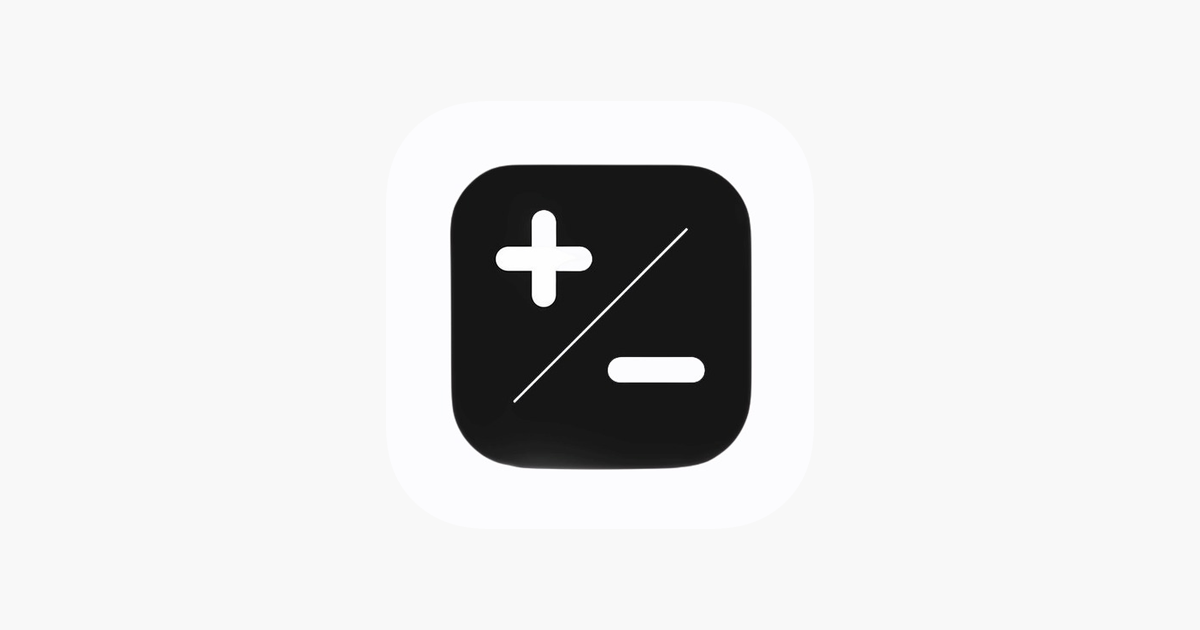# Calc+ – OnePercentCalc+ allows you to easily handle all the calculations necessary for everyday life with a single application.
A calculator app with an interactive graphical user interface

Features :

1. General Calculator
– Calculator which enables you to do simple and advanced calculations with ease.
– Display the result either in Standard form or Scientific for based on your requirements.
– Have the freedom to choose the number of decimals for accuracy depending on the purpose of the calculation.
– Stores all previous calculations in History locally.

2. Percentage Calculator
– Perform Percentage based calculations with ease without having to remember formulas.

3. BMI Calculator
– Calculate the BMI (Body Mass Index) of a person with the touch of a button.

4. Investment Calculator
– Calculate the Return of your Investment with the rate of interest being yearly, half yearly, quarterly, monthly or daily.

5. EMI and Loan Calculator
– Calculate your EMI and the total Interest payable.

6. Tax (VAT / GST) Calculator
– Get a total price by entering the original price and the tax rate.

7. Length Conversion
– Convert between Length units supporting all major Length Units such as Kilometer, Meter, Decimeter, Centimeter, Millimeter, Micrometer, Nanometer, Picometer, Nautical Mile, Mile, Yard, Foot, Inch, Lunar Distance, Astronomical Unit, Light Year.

8. Area Conversion
– Convert between Area units supporting all major Area Units such as Square Kilometer, Square Meter, Hectare, Square Decimeter, Square Centimeter, Square Millimeter, Square Micron, Acre, Square Mile, Square Yard. Square Yard, Square Foot, Square Inch, Square Rod.

9. Volume Conversion
– Convert between Volume units supporting all major Volume Units such as Liter, Hectoliter, Deciliter, Centiliter, Milliliter, Cubic Meter, Cubic Decimeter, Cubic Centimeter, Cubic Millimeter, Cubic Foot, Cubic Inch, Cubic Yard, Acre Foot.

10. Temperature Conversion
– Convert between Temperature units supporting all major Temperature Units such as Celsius, Fahrenheit, Kelvin, Rankine, Réamur.

11. Speed Conversion
– Convert between Speed units supporting all major Speed Units such as Lightspeed, Meter per second, Kilometer per second, Mile per hour, Kilometer per hour, Knot, Foot per second, Inch per second.

13. Time Conversion
– Convert between Time units supporting all major Time Units such as Century, Decade, Year, Week, Day, Hour, Minute, Second, Millisecond, Microsecond, Picosecond.

14. Mass Conversion
– Convert between Mass units supporting all major Mass Units such as Kilogram, Gram, Pounds, Tonne, Milligram, Microgram, Quintal, Ounce, Carat, Grain.

15. Numeral Conversion
– Convert between Numeral units supporting all major Numeral Units such as Binary, Octal, Decimal, Hexadecimal.

16. Quantity Conversion
– Convert between Quantity units supporting all major Quantity Units such as Liter, Gallon, Milliliter, Fluid Ounce, Cup, TableSpoon, TeaSpoon, Quart, Pint.

17. Date Calculator
– Calculate the difference between 2 dates in years, weeks, days, hours, minutes and seconds.

Website : www.OnePercent.club

Social Media :